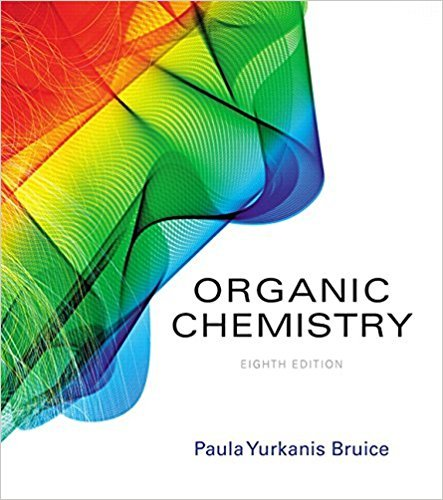×
Get Full Access to Organic Chemistry - 8 Edition - Chapter 8 - Problem 52
Get Full Access to Organic Chemistry - 8 Edition - Chapter 8 - Problem 52

×

# a. Predict the relative pKa values of cyclopentadiene and cycloheptatriene.b. PredictISBN: 9780134042282 482

## Solution for problem 52 Chapter 8

Organic Chemistry | 8th Edition

• Textbook Solutions
• 2901 Step-by-step solutions solved by professors and subject experts
• Get 24/7 help from StudySoup virtual teaching assistantsOrganic Chemistry | 8th Edition

4 5 1 305 Reviews
21
2
Problem 52

a. Predict the relative pKa values of cyclopentadiene and cycloheptatriene.b. Predict the relative pKa values of cyclopropene and cyclopropane.

Step-by-Step Solution:
Step 1 of 3

Gen Chem Notes:  Determining rate orders: ­ For this experiment we will solve for the rate orders. ­ To determine A, we will want to look for a spot where A changes but the value of B stays the same. Therefore, we may compare experiments 1 and 2. Between these experiments A doubles, while the rate quadruples. This means that A is a 2 order. ­ To determine B we may do the same with experiments 2 and 3. In this we see that B doubles, but the rate does not change. This means that B is zero order, for if the rate does not change while the concentration does then it is zero order. ­ Therefore, the total order of this reaction is 2, and the equation is rate = K [A]^2.  Once one has solved

Step 2 of 3

Step 3 of 3

##### ISBN: 9780134042282

The answer to “a. Predict the relative pKa values of cyclopentadiene and cycloheptatriene.b. Predict the relative pKa values of cyclopropene and cyclopropane.” is broken down into a number of easy to follow steps, and 19 words. Since the solution to 52 from 8 chapter was answered, more than 294 students have viewed the full step-by-step answer. This full solution covers the following key subjects: . This expansive textbook survival guide covers 127 chapters, and 2116 solutions. This textbook survival guide was created for the textbook: Organic Chemistry, edition: 8. Organic Chemistry was written by and is associated to the ISBN: 9780134042282. The full step-by-step solution to problem: 52 from chapter: 8 was answered by , our top Chemistry solution expert on 03/16/18, 04:59PM.

Unlock Textbook Solution